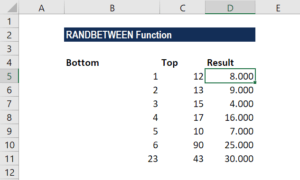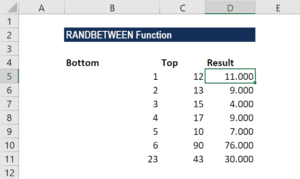# RANDBETWEEN Function

Returns a random integer number between the user-specified numbers

## What is the RANDBETWEEN Function?

The RANDBETWEEN Function is categorized under Excel Math and Trigonometry functions. The function will return a random integer number between the user-specified numbers. It will return a random integer number every time the worksheet is opened or calculated.

As a financial analyst, the RANDBETWEEN function can be used to generate random integer numbers within a specified range. However, it is used less frequently in the finance industry, as compared to other fields such as cryptography and statistics.

### Formula

=RANDBETWEEN(bottom,top)

The RANDBETWEEN function uses the following arguments:

1. Bottom (required function) – This is the smallest integer that the function will return.
2. Top (required function) – This is the largest integer that the function will return.

### How to use the RANDBETWEEN Function in Excel?

To understand the uses of the RANDBETWEEN function, let’s consider an example:

#### Example

When we enter data in the Bottom and Top columns below, we will get different results every time the worksheet is calculated or opened:The formula used was =RANDBETWEEN(B5,C5).

When we did some recalculations, the worksheet changed the results, as shown below:### Few notes about the RANDBETWEEN Function

1. RANDBETWEEN will calculate a new value each time the worksheet is calculated. If we wish to stop random numbers from being generated, we need to copy the cells that contain RANDBETWEEN to the clipboard and then use Paste Special > Values to convert them to text.
2. If we wish to get a random number that will not change when the worksheet is calculated, we need to enter the RANDBETWEEN function in the Formulas bar and then press F9 to convert the formula into its result.
3. If we wish to generate a set of random numbers in multiple cells, we need to select the cells, enter RANDBETWEEN function and press Ctrl + Enter after.

Thanks for reading CFI’s guide to this important Excel function. By taking the time to learn and master these Excel functions, you’ll significantly speed up your financial analysis. To learn more, check out these additional CFI resources:

• Excel Functions for Finance